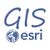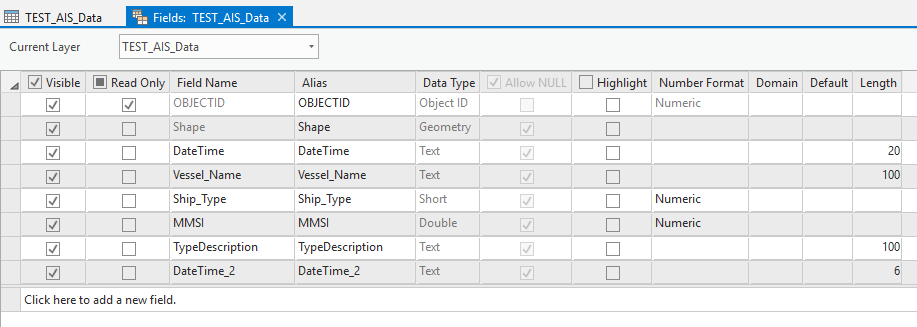# Why am I having another "Select" issue using a variable?

805
11
01-22-2020 04:17 PMOccasional Contributor II

I recently came to this forum with the virtually the same question, but now I'm using an "AND" instead of an "OR".  For the life of me I just cannot get this to work, and I don't know why.  The help I received for my last issue resolved my problem perfectly.  But now my query is only slightly more complex and it just won't go...so I'm wondering if a 2nd pair of eyes will help in my case.

It seems that I can do this when I hard code everything, but as soon as I try and use a variable it crashes with a very non-descript error message (e.g. Invalid Expression).

I have used 6 different expressions (listed and numbered below), and only expression2 and expression6 don't work...and these are the ones I need to work as this code is going to be transplanted into my main Python script once it's functioning.

expression1 is what works now after the help I received here...but I thought I could easily get the AND operator working because I now have the code working with an "OR" operator.  That didn't happen LOL.  Ideally, I would like to use the "AND" Boolean operator in this case (e.g. DateTime_2 = x and MMSI = y)...but I continue to struggle with this.

I try to keep my code very simple so it's easy for me to debug...and for the most part I'm able to do this (I'll continue to do this until I get better at Python scripting of course).  Can anyone see my errors?  Why can I not use expression2 or expression6?  I thought expression2 was almost an exact replica of expression1, but I guess not.

I use the exact same variable and value "record11" by itself in another script and it's fine...so I'm confident this isn't (by itself) the issue.

The query I would like to use would be similar to: DateTime_2 = fme_name_1b and MMSI = record11

``````import arcpy

year_1 = "2019"
month_1 = "01"
Q2_A = "201901"
record11 = 316003484

fme_name_1b = ((year_1) + (month_1)) #fme_name_1b now = 201901
print ("")
print ("fme_name_1b = " + str(fme_name_1b))
fc1 = r'F:\ArcGIS_Pro_Projects\AIS\AIS_Data\AIS_Data.gdb\TEST_AIS_Data'

#expression1 = ("DateTime_2 = '" + str(Q2_A) + "' or DateTime_2 = '" + str(Q2_B) + "' or DateTime_2 = '" + str(Q2_C) + "'") # WORKS PERFECTLY
#expression2 = ("DateTime_2 = '" + str(fme_name_1b) + "' and MMSI = '" + str(record11) + "'")  # DOES NOT WORK
#expression3 = ("DateTime_2 = '201907' And MMSI = 316012834") # WORKS PERFECTLY
#expression4 = ("DateTime_2 = '" + str(Q2_D) + "'") # WORKS PERFECTLY
expression5 = ("MMSI = 316003484") # WORKS PERFECTLY
#expression6 = ("MMSI = '" + str(record11) + "'") # DOES NOT WORK

print ("")
print (expression5)
print ("")
print ("Selecting records in fc1 that correspond to MMSI found in Summary Table")
SelectResult = arcpy.SelectLayerByAttribute_management((fc1), "NEW_SELECTION", (expression5))#Select records in feature class based on unique identifier (MMSI)
CountTotal = arcpy.GetCount_management((fc1))
CountResult = arcpy.GetCount_management(SelectResult)
print ("")
print (str(CountResult) + " Of " + str(CountTotal) + " Records Selected")‍‍‍‍‍‍‍‍‍‍‍‍‍‍‍‍‍‍‍‍‍‍‍‍‍‍‍‍‍‍‍‍‍‍‍‍‍‍‍‍‍‍‍‍‍‍‍‍‍‍‍‍‍‍‍‍‍‍‍‍‍‍‍‍‍‍‍‍‍‍‍‍‍‍‍‍‍‍‍‍‍‍‍‍‍‍‍‍‍‍‍‍‍‍‍‍‍‍‍‍‍‍‍‍‍‍‍‍‍‍‍‍‍‍‍‍‍‍‍‍‍‍‍‍‍‍‍‍‍‍‍‍‍‍‍‍‍‍‍‍‍‍‍‍‍‍‍‍‍‍‍‍‍‍‍‍‍‍‍‍‍‍‍‍‍‍‍‍‍‍‍‍‍‍‍‍‍‍‍‍‍‍‍‍``````

Thank you

Tags (2)
1 Solution

Accepted SolutionsOccasional Contributor II

Query Resolved:

I now have the script working.  What ultimately worked was:

record11 = 316003484

expression7 = ("MMSI = " + str(record11))

Removing the quotes from the variable was indeed what it took, but because I'm using the + symbol it kept balking until I made it a string (go figure).  So the Select Attributes does work now in spite of the fact that my variable is a string that contains a number, or the fact that this variable is used to query the MMSI field that is a double data type...and because of this one small hurdle am now able to add it to the larger query:

expression2 = ("DateTime_2 = '" + str(YearMonth) + "' and MMSI = " + str(record11))

printing each step

YearMonth = 201901

record11 = 316003484

expression2 = DateTime_2 = '201901' and MMSI = 316003484

Selecting records in fc1 based on expression

11 Of 75 Records Selected

I then tested this manually in ArcGIS Pro and I also end up with 11 of 75 records selected.  This portion of the script will now be added to the main program where it will query AIS data once per month that contains approximately 10-20 million records, filtered to 10%, and then published to Portal where heat maps of vessel traffic can be created.

Thanks for all of your help...the pointers I got here are all keepers and I will be reviewing all of this and doing a lot more reading :-).

11 RepliesbyMVP Legendary Contributor
`````` expression2 = ("DateTime_2 = '" + str(fme_name_1b) + "' and MMSI = '" + str(record11) + "'")  # DOES NOT WORK

expression2
"DateTime_2 = '201901' and MMSI = '316003484'"

expression3 = ("DateTime_2 = '201907' And MMSI = 316012834") # WORKS PERFECTLY

expression3
"DateTime_2 = '201907' And MMSI = 316012834"‍‍‍‍‍‍‍‍‍``````

the one that didn't work has '316003484' in single quotes...

the one that does work has 316003484 without quotes.

You might want to try doing what I did and print out the ones that work vs the fails to see the differencesbyMVP Legendary Contributor
`````` expression5 = ("MMSI = 316003484") # WORKS PERFECTLY
expression6 = ("MMSI = '" + str(record11) + "'") # DOES NOT WORK

expression5
'MMSI = 316003484'

expression6
"MMSI = '316003484'"‍‍‍‍‍‍‍‍``````

again a numeric vs a text representationbyMVP Esteemed Contributor

Regarding your overall question, i.e., why are you having another select issue, it seems you are struggling to understand the structure of SQL WHERE clauses, which is really where your issues lie.  Not to state the obvious, but it might be worth reading SQL reference for query expressions used in ArcGIS—ArcGIS Pro | ArcGIS Desktop and some other documentation on SQL WHERE clauses (Where (SQL) - Wikipedia )Occasional Contributor II

Thanks Joshua:

Admittedly I agree that I'm somewhat of a newbie to Python scripting and I do have a lot to learn.  However, my issue here is really not with the hard coding...I can get this query to work using the physical values.  The problem I'm having is getting the script to read the variable with those values that is being passed to that expression.

When the syntax is correct I also run into type issues as the program will typically crash with the error message:

ERROR 000358: Invalid expression

This "might" have something to do with data type, so my problem, I think, is learning more about quotation and how it works when using variables, plus how to pass non-strings to a variable.

To help illustrate where I'm at, I can't see the difference between:

("DateTime_2 = '201901' And MMSI = 316012834") - which works.

Or

fme_name_1b = '201901'

record11 = 316012834

("DateTime_2 = '" + str(fme_name_1b) + "' and MMSI = '" + str(record11) + "'")  - which does not work

the (record11) by itself won't work in a string with + symbology, as per:

expression6 = ('MMSI = "'(record11)'"')

The behavior of the script changes when one adds a variable...so how does one pass a numeric value in a variable to expression without adding quotes or converting the value to a string?

This part of the code works:  ("DateTime_2 = '" + str(fme_name_1b) + "'")

The program crashes only on this part: ("'MMSI = '" + str(record11) + "'") - or variations of which:

ExecuteError: ERROR 000358: Invalid expression
Failed to execute (SelectLayerByAttribute).

Suggestions?byMVP Legendary Contributor

look at my example for that case of record11  what is the data type for the field?Occasional Contributor II

Thanks again Dan:

I have tried variations of your example using a variable, but I run into syntax errors every time...I still don't see how this works.  Using "MMSI = '316003484'" works hard coded, but how should this look when using a variable?

"MMSI = '(record11)'" will (obviously) just turn the variable into a literal.

As mentioned above, my issue "might" be really about the data type.  In the file geodatabase MMSI is a "double":However, I haven't seen any data type options in Python for this...only "int", "float" and "str".  Why does a single quote work for the hard coded option then?  All I'm trying to do is "Select Attributes"...I'm not trying to calculate anything, so I would think that this should not be an issue...is it?

Is my alternative perhaps to change FME to convert that field into a string first (i.e. I don't know why it was originally coded to be a double as it's not really necessary - it's only an ID field)?byMVP Legendary Contributor

it if is numeric, you don't 'str' it

double/float/int are all numeric, so no single quotes around the numbers or the variable.

If the variable is somehow being cast as a string, then you will have to un-cast it by using 'float' around it instead of strOccasional Contributor II

Dan:

OK...I will play around with this code and see what I can do (knowing this).  The problem I believe I was having was trying to get the script to run without any quotes around the variable at all...but then ran into syntax issues.  There must be examples of how to do this properly online somewhere.byMVP Legendary Contributor

var = 123

"SomeField = {}".format(var)
'SomeField = 123'

var2 = "a string"

"SomeField = '{}'".format(var2)
"SomeField = 'a string'"

again, python mini formatting language